# Combining DataFrames with Pandas

• Last Updated : 11 Dec, 2020

Pandas DataFrame consists of three principal components, the data, rows, and columns. To combine these DataFrames, pandas provides multiple functions like concat() and append().

Method #1: Using concat() method

Attention geek! Strengthen your foundations with the Python Programming Foundation Course and learn the basics.

To begin with, your interview preparations Enhance your Data Structures concepts with the Python DS Course. And to begin with your Machine Learning Journey, join the Machine Learning - Basic Level Course

Initially, creating two datasets and converting them into dataframes.

## Python3

 `# import required module``import` `pandas as pd`` ` `# making  a dataset``data1 ``=` `{``    ``'Serial_No.'``: [``'1'``, ``'2'``, ``'3'``, ``'4'``, ``'5'``],``    ``'First'``: [``'F0'``, ``'F1'``, ``'F2'``, ``'F3'``, ``'F4'``],``    ``'Second'``: [``'S0'``, ``'S1'``, ``'S2'``, ``'S3'``, ``'S4'``],``}`` ` `# creating a dataframe``df1 ``=` `pd.DataFrame(data1, columns``=``[``'Serial_No.'``, ``                                   ``'First'``, ``                                   ``'Second'``])`` ` `# display dataframe``df1`` ` ` ` `# making  a dataset``data2 ``=` `{``    ``'Serial_No.'``: [``'6'``, ``'7'``, ``'8'``, ``'9'``, ``'10'``],``    ``'First'``: [``'F10'``, ``'F11'``, ``'F12'``, ``'F13'``, ``'F14'``],``    ``'Second'``: [``'S10'``, ``'S11'``, ``'S12'``, ``'S13'``, ``'S14'``],``}`` ` `# creating  a dataset``df2 ``=` `pd.DataFrame(data2, columns``=``[``'Serial_No.'``, ``                                   ``'First'``, ``                                   ``'Second'``])`` ` `# display dataset``df2`

Output: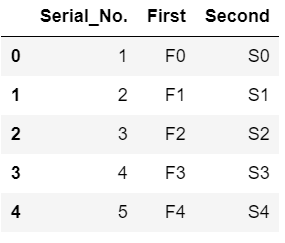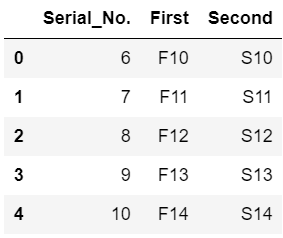Now, concatenating the two dataframes, we will use concat() to combine two dataframes. If ignore_index = True the index of df will be in a continuous order.

## Python3

 `# combining the two dataframes``df ``=` `pd.concat([df1, df2], ignore_index``=``True``)`` ` `# display combined dataframes``df`

Output: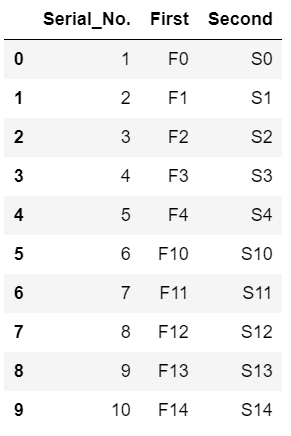Using keys we can specify the labels of the dataframes.

## Python3

 `# we can also separate 2 datasets using keys``frames ``=` `[df1, df2]``df_keys ``=` `pd.concat(frames, keys``=``[``'x'``, ``'y'``])`` ` `# display dataframe``df_keys`

Output: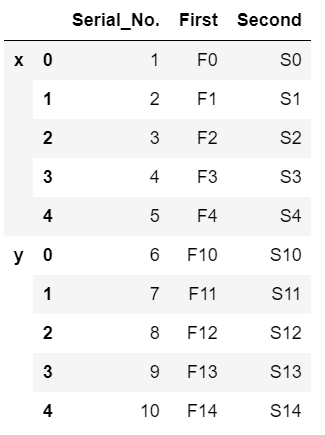Method #2: Using append() method

Initially, creating two datasets and converting them into dataframes.

## Python3

 `# import required module``import` `pandas as pd`` ` `# making  a dataset``data1 ``=` `{``    ``'Serial_No.'``: [``'1'``, ``'2'``, ``'3'``, ``'4'``, ``'5'``],``    ``'First'``: [``'F0'``, ``'F1'``, ``'F2'``, ``'F3'``, ``'F4'``],``    ``'Second'``: [``'S0'``, ``'S1'``, ``'S2'``, ``'S3'``, ``'S4'``],``}`` ` `# creating a dataframe``df1 ``=` `pd.DataFrame(data1, columns``=``[``'Serial_No.'``, ``                                   ``'First'``, ``                                   ``'Second'``])`` ` `# display dataframe``df1`` ` ` ` `# making  a dataset``data2 ``=` `{``    ``'Serial_No.'``: [``'6'``, ``'7'``, ``'8'``, ``'9'``, ``'10'``],``    ``'First'``: [``'F10'``, ``'F11'``, ``'F12'``, ``'F13'``, ``'F14'``],``    ``'Second'``: [``'S10'``, ``'S11'``, ``'S12'``, ``'S13'``, ``'S14'``],``}`` ` `# creating  a dataset``df2 ``=` `pd.DataFrame(data2, columns``=``[``'Serial_No.'``, ``                                   ``'First'``, ``                                   ``'Second'``])`` ` `# display dataset``df2`

Output:The dataframe.append() method performs the operation of combining two dataframes similar to that of the contcat() method.

## Python3

 `# combining dataframes``result ``=` `df1.append(df2, sort``=``False``, ignore_index``=``True``)`` ` `# display combined dataframe``result`

Output: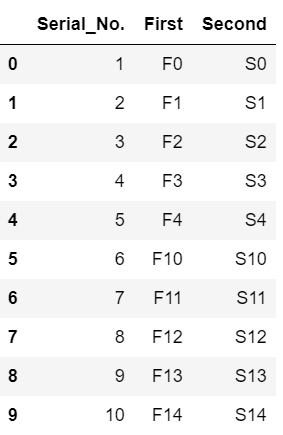My Personal Notes arrow_drop_up